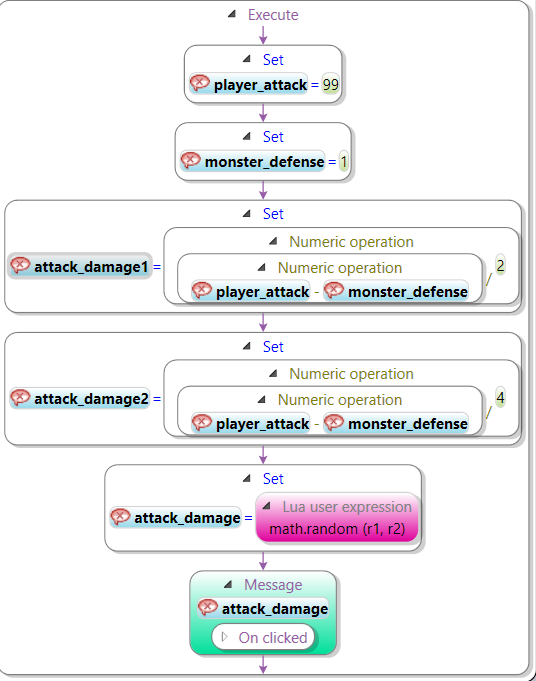# Combat Math Formula

Followers 1

## Recommended Posts

I am creating an RPG style Wherigo where I need to calculate attack damage using the formula:

attack_var1 (r1) = (player_attack - monster_defense) / 2

attack_var2 (r2) = (player_attack - monster_defense) / 4

attack_damage = math.random (r1, r2)

I would like to display the damage as a range of values between the two attack variables. When I create this code in urwigo I keep getting an error message. Does anyone know how to make this formula work in urwigo? I will upload a pic of what I have.

Btw, I set the identifier for attack_var1 and attack_var2 as "r1" and "r2."Posted (edited)

Double check your math! attack_damage1 is divided by 2, while attack_damage2 is divided by 4. Thus, attack_damage1 is greater than (or equal to) attack_damage2. So, when you call math.random(r1, r2), you trip a "interval is empty" exception (recall that math.random selects a random integer greater than or equal to the first argument and lesser than or equal to the second argument. So, if r1 is greater than r2, then the "interval is empty.")

The solution should be as easy as changing math.random(r1, r2) to math.random(r2, r1).

(And, some advice to avoid this again—consider renaming "attack_damage1" to "upper_bound" and "attack_damage2" to "lower_bound". That way, it's obvious without even thinking about it.)

Edited by Hügh
•1

That fixed it!!! Thank you so much. I spent a really long time going back and forth over my code but could not figure it out. I didn't know how the rules of math.random work so I learned something here too. I will rename the variable identifiers to upper and lower bounds as well. Thank you again!

## Join the conversation

You can post now and register later. If you have an account, sign in now to post with your account.
Note: Your post will require moderator approval before it will be visible.×   Pasted as rich text.   Paste as plain text instead

Only 75 emoji are allowed.